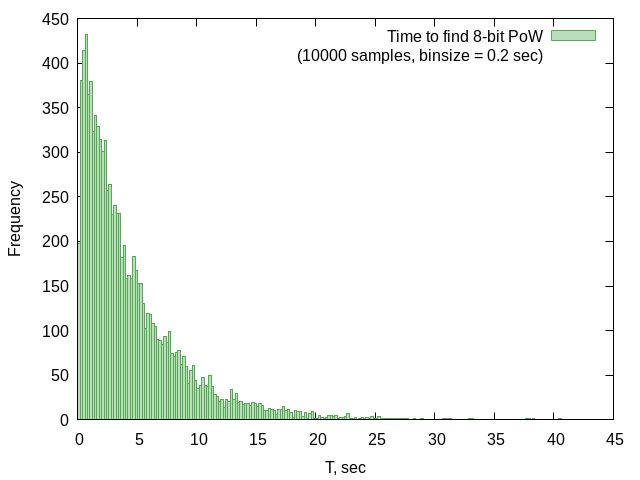## Purpose

Proof-of-work (PoW) in Dscuss is used for resistance against flooding. PoW is just a `uint64` number, which is sent along with users' public key. Hash of user's public key plus PoW must has a determined number of leading zero bits (see below). It should be hard to find such a PoW. Hence the selected hashing function is scrypt. Peers are forced to do this work before participating in discussion, otherwise their messages will be dropped.

## Math

Average time to find a PoW is

``````Tavg = C * 2 ^ n
``````

Where C is a constant, which depends on peer's CPU performance and n is the number of leading zeros in the resulting hash.

The time to find PoW (T) is actually a random variable, which has a geometric distribution. Tavg is the expected value of this variable. The real distribution of time to find an 8-bit (as an example) PoW is shown on the histogram below. The histogram is drawn for 10,000 values.The number of required leading zero bits is set to `16` for the proof-of-concept version. In this case, Tavg is about 20 minutes on ```Intel(R) Core(TM) i7-4790k CPU @ 4.0GHz``` or 2 hours on ```Intel(R) Core(TM) 2 Duo P8600 @ 2.40GHz```.

## Benchmarking

How to benchmark proof-of-work on your system:

1. Disable `debug` in dscuss.go.
2. Run `utils/benchmark_pow.sh [bit_num] [count]`
where,
`bit_num` is the number of leading zero bits in PoW (can be 8 or 16);
`count` specifies how many times to run the test.

for example:

``````utils/benchmark_pow.sh 16 50
``````

This test takes about 12 hours on Intel Core i7-4790k.

When the benchmark is over, you will see the results: the Tavg and the histogram:

``````The benchmark is over.
The average time to find proof is: 22.2161
In `benchmark.log` you will find details about each test run.